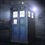# A simple proof?

Given:$a=b\times q+r,0\leq r < b$.Yes,that is the division equation.Try to prove that,$GCD(a,b)=GCD(b,r)$.Try not to use any theorem for solving this.Just use simple Algebra.This is from my class $10^{th}$ textbook,it was just written as a statement and no proof was given.I came up with one which I will post afterwards.Note by Adarsh Kumar
6 years, 2 months ago

This discussion board is a place to discuss our Daily Challenges and the math and science related to those challenges. Explanations are more than just a solution — they should explain the steps and thinking strategies that you used to obtain the solution. Comments should further the discussion of math and science.

When posting on Brilliant:

• Use the emojis to react to an explanation, whether you're congratulating a job well done , or just really confused .
• Ask specific questions about the challenge or the steps in somebody's explanation. Well-posed questions can add a lot to the discussion, but posting "I don't understand!" doesn't help anyone.
• Try to contribute something new to the discussion, whether it is an extension, generalization or other idea related to the challenge.
• Stay on topic — we're all here to learn more about math and science, not to hear about your favorite get-rich-quick scheme or current world events.

MarkdownAppears as
*italics* or _italics_ italics
**bold** or __bold__ bold
- bulleted- list
• bulleted
• list
1. numbered2. list
1. numbered
2. list
Note: you must add a full line of space before and after lists for them to show up correctly
paragraph 1paragraph 2

paragraph 1

paragraph 2

[example link](https://brilliant.org)example link
> This is a quote
This is a quote
    # I indented these lines
# 4 spaces, and now they show
# up as a code block.

print "hello world"
# I indented these lines
# 4 spaces, and now they show
# up as a code block.

print "hello world"
MathAppears as
Remember to wrap math in $$ ... $$ or $ ... $ to ensure proper formatting.
2 \times 3 $2 \times 3$
2^{34} $2^{34}$
a_{i-1} $a_{i-1}$
\frac{2}{3} $\frac{2}{3}$
\sqrt{2} $\sqrt{2}$
\sum_{i=1}^3 $\sum_{i=1}^3$
\sin \theta $\sin \theta$
\boxed{123} $\boxed{123}$

## Comments

Sort by:

Top Newest

To prove :- If $a=bq+r$, then $(a,b)=(b,r)$, where $(x,y)$ denotes GCD of x and y. Proof:- Let $(a,b)=d$ and $(b,r)=e$. Then, (a,b) =$d \Rightarrow d|a$ and $d|b \Rightarrow d|a$ and $d|qb \Rightarrow d|(a-qb) \Rightarrow d|r$. Hence, $d|r$ and $d|b \Rightarrow \boxed{d|(b,r)}$. Similarly, (b,r) = $e \Rightarrow e|b$ and $e|r \Rightarrow e|bq$ and $e|r \Rightarrow e|(bq-r) \Rightarrow e|a$. Hence, $e|a$ and $e|b \Rightarrow \boxed{e|(a,b)}$. We see from the boxed expressions that $(a,b)|(b,r)$ and $(b,r)|(a,b) \Rightarrow (a,b) = (b,r)$. Q.E.D.

- 6 years, 2 months ago

Log in to reply

I completed the proof in the same way - I personally remember the proof from "Elementary Number Theory " by Jones - It's a great book :)

- 6 years, 2 months ago

Log in to reply

Thanks a lot for your suggestion sir 👍 ! The book is really awesome for beginners 💎 !

- 6 years, 2 months ago

Log in to reply

Very nice proof!But how did you come up with it so fast?Have you seen similar problems before?

- 6 years, 2 months ago

Log in to reply

Yeah, I am pretty familiar with these kind of problems ! I came across problems involving proofs of gcd while doing number theory...

- 6 years, 2 months ago

Log in to reply

So,is this your own?Do u have any NT books?

- 6 years, 2 months ago

Log in to reply

Not my own bro, I just remember seeing this proof in some book ! Btw this is really important one, it is the basis of the Euclids Algorithm ! For good books, check out David A. Santos books, they are available in pdf format freely, and they are really good books to follow 🎓 📱 !

- 6 years, 2 months ago

Log in to reply

Yes,yes this is really an important one!It is the basis of Euclid's Algorithm,that is how I came across this.It must be in your book also.Check it!

- 6 years, 2 months ago

Log in to reply

Oh!Thanx for the recommendations!Thanx a lot!!Grateful!

- 6 years, 2 months ago

Log in to reply

×

Problem Loading...

Note Loading...

Set Loading...